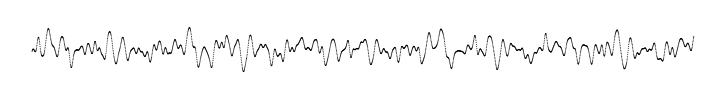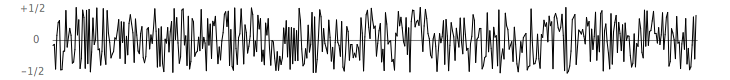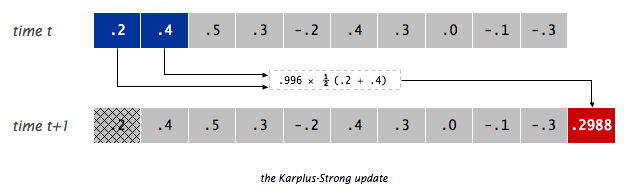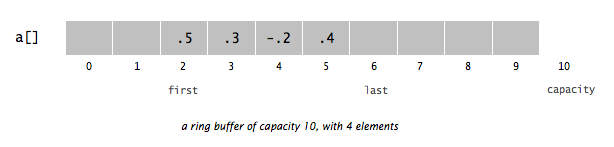COS 126 Plucking a Guitar String Programming Assignment Due: 11:55pm

Write a program to simulate plucking a guitar string using the Karplus-Strong algorithm. This algorithm played a seminal role in the emergence of physically modeled sound synthesis (where a physical description of a musical instrument is used to synthesize sound electronically).

Digital audio. Before reading this assignment, review the material in the textbook on digital audio (pp. 147-151, 202-206).

Simulate the plucking of a guitar string. When a guitar string is plucked, the string vibrates and creates sound. The length of the string determines its fundamental frequency of vibration. We model a guitar string by sampling its displacement (a real number between -1/2 and +1/2) at N equally spaced points (in time), where N equals the sampling rate (44,100) divided by the fundamental frequency (rounded to the nearest integer).• Plucking the string. The excitation of the string can contain energy at any frequency. We simulate the excitation by filling the buffer with white noise: set each of the N sample displacements to a random real number between -1/2 and +1/2.• The resulting vibrations. After the string is plucked, the string vibrates. The pluck causes a displacement which spreads wave-like over time. The Karplus-Strong algorithm simulates this vibration by maintaining a ring buffer of the N samples: the algorithm repeatedly deletes the first sample from the buffer and adds to the end of the buffer the average of the first two samples, scaled by an energy decay factor of 0.996.Why it works? The two primary components that make the Karplus-Strong algorithm work are the ring buffer feedback mechanism and the averaging operation.

• The ring buffer feedback mechanism. The ring buffer models the medium (a string tied down at both ends) in which the energy travels back and forth. The length of the ring buffer determines the fundamental frequency of the resulting sound. Sonically, the feedback mechanism reinforces only the fundamental frequency and its harmonics (frequencies at integer multiples of the fundamental). The energy decay factor (.996 in this case) models the slight dissipation in energy as the wave makes a roundtrip through the string.

• The averaging operation. The averaging operation serves as a gentle low pass filter (which removes higher frequencies while allowing lower frequencies to pass, hence the name). Because it is in the path of the feedback, this has the effect of gradually attenuating the higher harmonics while keeping the lower ones, which corresponds closely with how actually plucked strings sound.

Ring buffer. Your first task is to create a data type to model the buffer. Write a class named RingBuffer that implements the following API.

```        RingBuffer(int capacity)  // constructor to create an empty buffer, with given max capacity
int size()                    // return number of items currently in the buffer
boolean isEmpty()                 // is the buffer empty (size equals zero)?
boolean isFull()                  // is the buffer full  (size equals capacity)?
void enqueue(double x)         // add item x to the end
double dequeue()                 // delete and return item from the front
double peek()                    // return (but do not delete) item from the front
```
Since the buffer has a known maximum capacity, you can implement a RingBuffer using a double array of that length. For efficiency, use cyclic wrap-around: Maintain one integer instance variable first that stores the index of the least recently inserted item; maintain a second integer instance variable last that stores the index one beyond the most recently inserted item. To insert an item, put it at index last and increment last. To remove an item, take it from index first and increment first. When either index equals capacity, make it wrap-around by changing the index to 0.Guitar string. Next, write a data type to model a vibrating guitar string of a given frequency. Implement a class named GuitarString with the following API.

```       GuitarString(double frequency)
GuitarString(double[] init)
void pluck()                         // set the buffer to white noise
void tic()                           // advance the simulation one time step
double sample()                        // return the current sample
int time()                          // return number of tics
```

• Constructors. There are two ways to create a GuitarString object.

• The first constructor creates a RingBuffer of the desired capacity N (sampling rate divided by frequency, rounded to the nearest integer), and initializes it to represent a guitar string at rest by enqueueing N zeros.

• The second constructor creates a RingBuffer of capacity equal to the size of the array, and initializes the contents of the buffer to the values in the array. On this assignment, its purpose is solely for debugging.

• Pluck. Replace the N elements in the buffer with N random values between -0.5 and +0.5.

• Tic. Apply the Karplus-Strong update: delete the sample at the front of the buffer and add to the end of the buffer the average of the first two samples, multiplied by the energy decay factor.

• Sample. Return the value of the item at the front of the buffer.

• Time. Return the total number of times tic() was called.

Interactive guitar player. Here is a sample client that plays the guitar in real-time, using the keyboard to input notes. When the user types the uppercase letter 'A' or 'C', the program plucks the corresponding string. Since the combined result of several sound waves is the sum of the individual sound waves, we play the sum of all string samples.

 ``` public class GuitarHeroLite { public static void main(String[] args) { // create two guitar strings, for concert A and C double CONCERT_A = 440.0; double CONCERT_C = CONCERT_A * Math.pow(2, 3.0/12.0); GuitarString stringA = new GuitarString(CONCERT_A); GuitarString stringC = new GuitarString(CONCERT_C); while (true) { // check if the user has typed a key; if so, process it if (StdDraw.hasNextKeyTyped()) { char key = StdDraw.nextKeyTyped(); if (key == 'A') { stringA.pluck(); } else if (key == 'C') { stringC.pluck(); } } // compute the superposition of samples double sample = stringA.sample() + stringC.sample(); // send the result to the sound card StdAudio.play(sample); // advance the simulation of each guitar string by one step stringA.tic(); stringC.tic(); } } } ```
Write a program GuitarHero that is similar to GuitarHeroLite, but support a total of 37 notes on the chromatic scale from 110Hz to 880Hz. In general, make the ith character of the string
```String keyboard = "1234567890qwertyuiopasdfghjklzxcvbnm,";
```
correspond to a frequency of 440 × 2(i - 24) / 12, so that the character '1' is 110Hz, 'e' is 220Hz, 'g' is 440Hz, and ',' is 880Hz. Don't even think of including 37 individual GuitarString variables or a 37-way if statement! Instead, create an array of 37 GuitarString objects and use keyboard.indexOf(key) to figure out which key was typed.

Deliverables. Submit RingBuffer.java, GuitarString.java, and GuitarHero.java along with a readme.txt file.

Extra credit 1. Write a program GuitarHeroVisualizer.java (by modifying GuitarHero.java) that plots the sound wave in real-time, as the user is playing the keyboard guitar. The output should look something like this, but change over time.Extra credit 2. Modify the Karplus-Strong algorithm to synthesize a different instrument. Consider changing the excitation of the string (from white-noise to something more structured) or changing the averaging formula (from the average of the first two samples to a more complicated rule) or anything else you might imagine.

Extra credit 3. Bring your laptop to precept and perform a piece to your classmates.

This assignment was created by Andrew Appel, Jeff Bernstein, Maia Ginsburg, Ken Steiglitz, Ge Wang, and Kevin Wayne.